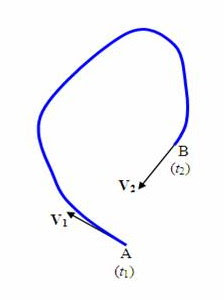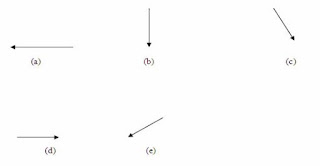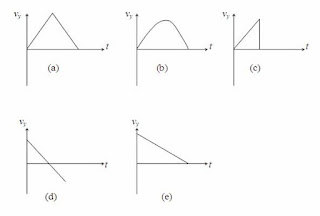## Pages

`“Life is like riding a bicycle.  To keep your balance you must keep moving.”–Albert Einstein`

## Saturday, March 24, 2012

### AP Physics B & C - Multiple Choice Practice Questions on Motion in Two Dimensions including Projectile Motion

"If man is not rising upward to be an angel, he is sinking downward to be a devil. He cannot stop at the beast."

– Samuel Taylor Coleridge

Let us discuss a few questions (MCQ) involving motion in two dimensions. Questions in common for AP Physics B as well as AP Physics C aspirants are discussed first. Questions specifically meant for AP Physics C aspirants are discussed next.(1) The adjoining figure represents the path of an ant on a horizontal floor between the instants t1 and t2. The velocities of the ant at positions A an B at the instants t1 and t2 are represented by the vectors V1 and V2 respectively. Which one among the following vectors best represents the net acceleration of the ant during the interval t2 t1?The acceleration a is given by

a = (V2 V1)/(t2t1)

The direction of the vector V2 V1 is given correctly in option (b).

[To find V2 V1 you have to add the vector V2 to the vector –V1 using the parallelogram law (for convenience). On reversing the direction of V1 and using it along with V2 to form the parallelogram, you get the diagonal which points along the vector shown in option (b)].

The correct option is indeed (b).(2) The path of a particle projected from point A with velocity v at an angle θ with respect to the horizontal is shown in the adjoining figure. Which one among the following graphs best represents the vertical component (vy) of the velocity of the particle as a function of time t? (Assume that air resistance is negligible)Since the motion of the particle is under gravity, the vertical component of velocity decreases uniformly as the particle moves up. At maximum height the vertical component of velocity momentarily becomes zero. Then the particle moves down and the vertical component of velocity goes on increasing uniformly until it hits the ground. The correct graph is (d).

[Note that the upward direction of the vertical component of velocity is taken as positive and the downward direction is taken as negative].

(3) In the above question, if g represents the gravitational acceleration, the values of acceleration of the particle at points A, B and C of the trajectory are

(a) greater than g, zero and less than g respectively

(b) greater than g, equal to g and less than g respectively

(c) less than g, zero and greater than g respectively

(d) less than g, equal to g and greater than g respectively

(e) same and equal to g

The gravitational force is the same everywhere along the trajectory and hence the acceleration of the particle is the same (equal to g).

The correct option is (e).

The following questions are specifically meant for AP Physics C aspirants:

(4) A particle starts from the origin at time t = 0 with velocity 2 ĵ and moves in the x-y plane with a constant acceleration of 2 î + 4 ĵ where î and ĵ are unit vectors along the x-direction and y irection respectively. What will be the x-coordinate of the particle when its y-coordinate becomes 12 m?

(a) 4 m

(b) 6 m

(c) 6.8 m

(d) 8 m

(e) 12 m

The position vector rt of the particle at the instant t is given by

rt = v0t + ½ at2 where v0 is the initial velocity and a is the constant acceleration.

We have v0 = 2 ĵ and a = 2 î + 4 ĵ

Therefore, rt = 2 ĵ t + (½) (2 î + 4 ĵ)t2 = t2 î + (2t + 2t2) ĵ

The above equation shows that the x-coordinate of the particle at time t is t2 and the y-coordinate is (2t + 2t2)

The time t at which the y-coordinate becomes 12 metre is given by

2t + 2t2 = 12

Or, 2t2 + 2t 12 = 0

This gives t = [–2 ±√(4 + 96)]/4 = 2 seconds, ignoring the negative time.

Since the x-coordinate of the particle is t2, its value when the y-coordinate becomes 12 m (at time 2 seconds) is 4 m [Option (a)].

(5) What is the speed of the particle in the above question at the time t = 1 s?

(a) 2 ms–1

(b) √(10) ms–1

(c) √(20) ms–1

(d) √(40) ms–1

(e) 8 ms–1

The position vector rt of the particle at time t, as shown above is given by

rt = t2 î + (2t + 2t2) ĵ

The velocity vt of the particle at time t is given by

vt = drt/dt = 2t î + (2 + 4t) ĵ

When the time t = 1 s, the velocity of the particle is 2 î + 6 ĵ.

The speed of the particle at time 1 s is the magnitude of the above velocity and is equal to √(22 + 62) = √(40) m

You will find a useful post in this section here.